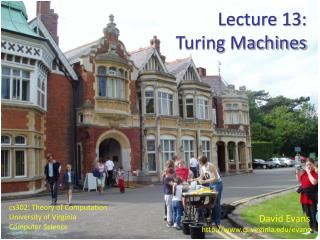DownloadDownload PresentationDavid Evans http://www.cs.virginia.edu/evans

# David Evans http://www.cs.virginia.edu/evans

Download Presentation## David Evans http://www.cs.virginia.edu/evans

- - - - - - - - - - - - - - - - - - - - - - - - - - - E N D - - - - - - - - - - - - - - - - - - - - - - - - - - -
##### Presentation Transcript

1. Lecture 13: Turing Machines David Evans http://www.cs.virginia.edu/evans cs302: Theory of Computation University of Virginia Computer Science

2. Violates Pumping Lemma For CFLs The Story So Far Indexed Grammars 0n1n2n 0n1n Violates Pumping Lemma For RLs 0n Described by DFA, NFA, RegExp, RegGram w Described by LL(k) Grammar Described by CFG, NDPDA Regular Languages Deterministic CFLs LL(k) Languages Context-Free Languages

3. Violates Pumping Lemma For CFLs The Story So Far (Simplified) 0n1n Violates Pumping Lemma For RLs 0n Described by DFA, NFA, RegExp, RegGram w Described by CFG, NDPDA Regular Languages Context-Free Languages

4. Computability Story: This Week Languages recognizable by any mechanical computing machine

5. Computability Story: Next Week Undecidable Problems Decidable Problems Recognizable Languages

6. Computability  Complexity (April) Decidable Problems Decidable Problems P NP Note: not known if P  NP or P = NP Problems that can be solved by a computer (eventually). Problems that can be solved by a computer in a reasonable time.

7. Exam 1

8. Exam 1 • Problem 4c: Prove that the language {0n1n2} is not context-free. Pumping lemma for CFLs says there must be some way of picking s = uvxyz such that m = |v| + |y| > 0 and uvixyizinLfor alli. So, increasing i by 1 adds m symbols to the string, which must produce a string of a length that is not the length of a string in L. Lengths of strings in L: n = 0 0 + 02 = 0 n = 1 1 + 12 = 2 n = 2 2 + 22 = 6 n = 3 3 + 32 = 12 ... n = k k + k2

9. Recognizing {0n1n2} DPDA with two stacks? DPDA with three stacks? ... ?

10. 3-Stack DPDA Recognizing {0n1n2} 0, ε/ε/ε\$/\$/\$ 0, ε/ε/ε+/ε/+ Count 0s Start 1, +/ε/εε/+/ε 1, +/\$/+ε/+/ε 1, \$/\$/\$ε/ε/ε 1s b->r 1, \$/+/+\$/ε/ε 1, \$/ε/\$ε/ε/ε 1s r->b 1, +/ε/εε/+/ε Done 1, ε/+/ε+/ε/ε 1, ε/\$/\$ε/ε/ε

11. Can it be done with 2 Stacks?

12. Simulating 3-DPDA with 2-DPDA + pushB(\$) pushB(popA()) ... pushB(popA(#)) pushB(popA()) ... pushB(popA(#)) res = popA() pushA(popB(#)) pushA(popB()) ... popB(\$) # # pop green \$ 3-DPDA B A

13. 2-DPDA + Forced ε-Transitions Need to do lots of stack manipulation to simulate 3-DPDA on one transition: need transitions with no input symbol (but not nondeterminism!) # # \$ B A

14. Impact of Forced ε-Transitions What is the impact of adding non-input consuming transitions? # DPDA in length n input: runs for  n steps DPDA+ε in length n input: can run forever! # \$ B A

15. What about an NDPDA? Use one stack to simulate the NDPDA’s stack. Use the other stack to keep track of nondeterminism points: copy of stack and decisions left to make. B A

16. Turing Machine? Tape Head B A

17. Turing Machine . . . FSM Infinite tape: Γ* Tape head: read current square on tape, write into current square, move one square left or right FSM: like PDA, except: transitions also include direction (left/right) final accepting and rejecting states

18. Turing Machine Formal Description . . . FSM 7-tuple: (Q, , Γ, δ, q0, qaccept, qreject) Q: finite set of states : input alphabet (cannot include blank symbol, _) Γ: tape alphabet, includes  and _ δ: transition function:Q Γ QΓ {L, R} q0: start state, q0 Q qaccept: accepting state, qaccept Q qreject: rejecting state, qreject Q (Sipser’s notation)

19. Turing Machine Computing Model Initial configuration: x . . . x x x x x x x _ _ _ _ blanks input FSM q0 TM Configuration: Γ* QΓ* tape contents left of head tape contents head and right current FSM state

20. TM Computing Model δ*: Γ* QΓ*  Γ* QΓ* The qaccept and qreject states are final: δ*(L, qaccept, R) (L, qaccept, R) δ*(L, qreject, R) (L, qreject, R)

21. FSM q TM Computing Model δ*: Γ* QΓ*  Γ* QΓ* u, v Γ*, a, bΓ . . . a b v u δ*(ua, q, bv)= (uac, qr, v) if δ(q, b) = (qr, c, R) δ*(ua, q, bv)= (u, qr, acv) if δ(q, b) = (qr, c, L) Also: need a rule to cover what happens at left edge of tape

22. Thursday’s Class Read Chapter 3: It contains the most bogus sentence in the whole book. Identify it for +25 bonus points (only 1 guess allowed!) • Robustness of TM model • Church-Turing Thesis